# Creating Trading Strategies and Backtesting with R

Updated: Oct 30, 2020

### Create fully functional trading strategies and backtest the results with R and its powerful librariesClick here to view this article on Medium (US Based online publishing platform)

R Programming language is an open-source software developed by statisticians and it is widely used among Data Miners for developing Data Analysis. R can be best programmed and developed in RStudio which is an IDE (Integrated Development Environment) for R. The advantages of R programming language includes quality plotting and eye-catching reports, a vast source of packages used for various functions, the exemplary source for Data Wrangling, the performance of various Machine Learning operations and flashy for Statistics. In my experience, R is the best language for self-learning and also it is very flexible for Financial Analysis and Graphing.

In this article, we are going to create six trading strategies and backtest its results using R and its powerful libraries. (Note: All codes are written in RStudio IDE, you can prefer other IDEs too)

## Steps Involved in this process

1. Importing required libraries

2. Extracting stock data (Apple, Tesla, Netflix) from Yahoo and Basic Plotting

3. Creating technical indicators

4. Creating trading signals

5. Creating trading strategies

6. Backtesting and Comparing the results

### Step-1: Importing required libraries

Firstly, to perform our process of creating trading strategies, we have to import the required libraries to our environment. Throughout this process, we will be using some of the most popular finance libraries in R namely Quantmod, TTR, and Performance Analytics. Using the library function in R, we can import our required packages. Let's do it!

Yes! We successfully completed our first step!

### Step-2: Extracting Data from Yahoo and Basic Plotting

Throughout our process, we will be working with the stock price data of Apple, Tesla, and Netflix. Let's extract the data of these companies from Yahoo in R

Now let's do some visualization of our extracted data! The following code produces a financial bar chart of stock prices along with volume.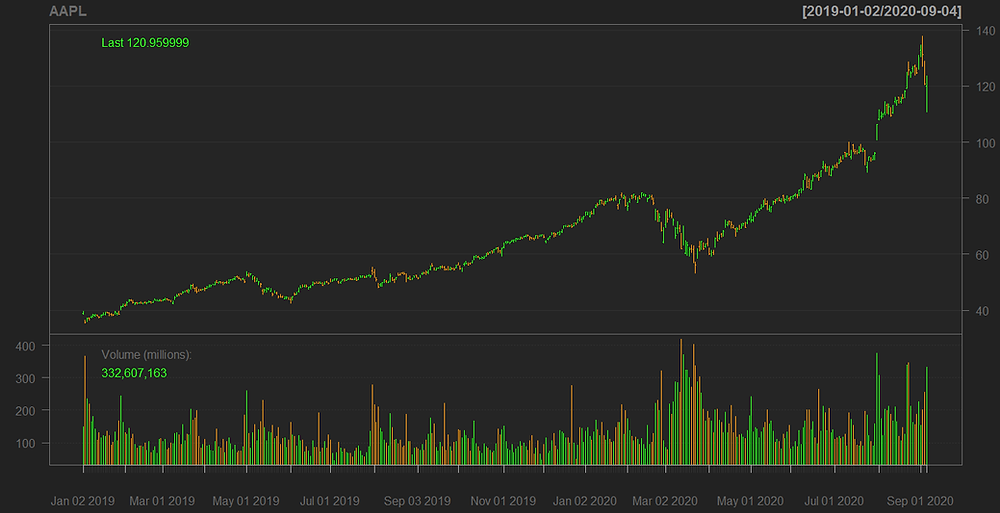### Step-3: Creating Technical Indicators

There are many technical indicators used for financial analysis but, for our analysis, we are going to use and create six of the most famous technical indicators namely: Simple Moving Average (SMA), Parabolic Stop And Reverse (SAR), Commodity Channel Index (CCI), Rate Of Change (ROC), Stochastic Momentum Index (SMI) and finally Williams %R. Let's do it!

Simple Moving Average (SMA) :

The standard interval of time we are going to take is 20 days SMA and 50 days SMA. But, there no restrictions to use any interval of time.

The following code will calculate three companies' 20 days SMA and 50 days SMA along with a plot:Parabolic Stop And Reverse (SAR) :

To calculate Parabolic SAR, we have to pass on the daily High and Low prices of the companies along with a given acceleration value.

The following code will calculate the companies' Parabolic SAR along with a plot:Commodity Channel Index (CCI) :

To calculate CCI, we have to pass on the daily High, Low, and Close prices of companies along with a specified time period and a constant value. In this step, we are going to take 20 days as the time period and 0.015 as the constant value.

The following code will calculate the companies' CCI along with a plot: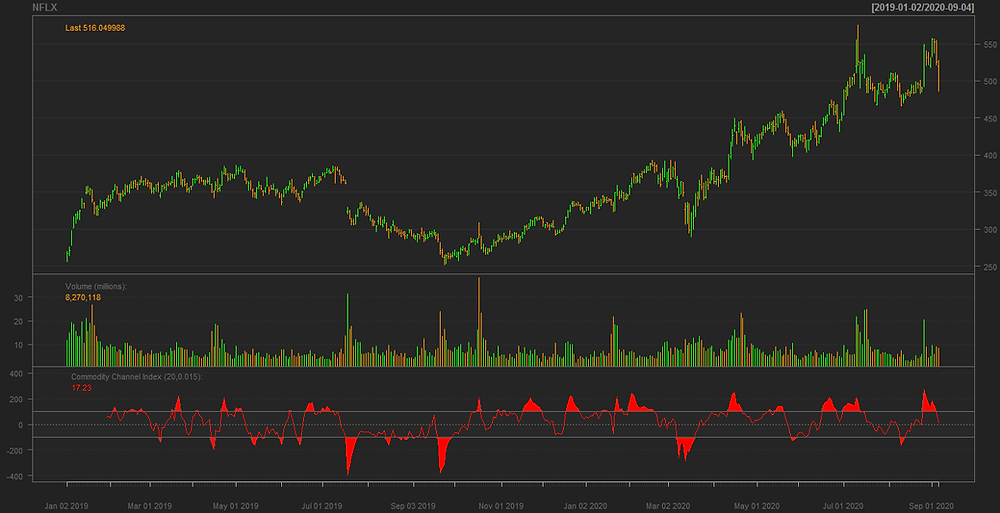Rate Of Change (ROC)

To calculate ROC, we have to pass on a specified interval of time and there are no restrictions in using any period. In this step, we are going to take 25 days as a period of time.

The following code will calculate the companies' ROC along with a plot: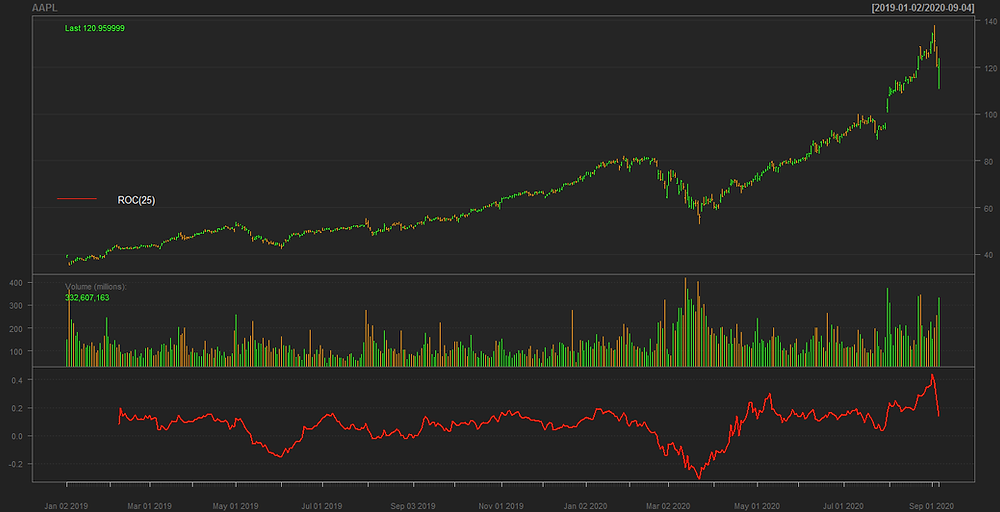Stochastic Momentum Index (SMI) :

To calculate SMI, we have to pass on the daily High, Low, and Close prices of companies, a specified interval of time, two smoothing parameters, and a signal value.

The following code will calculate companies' SMI along with a plot: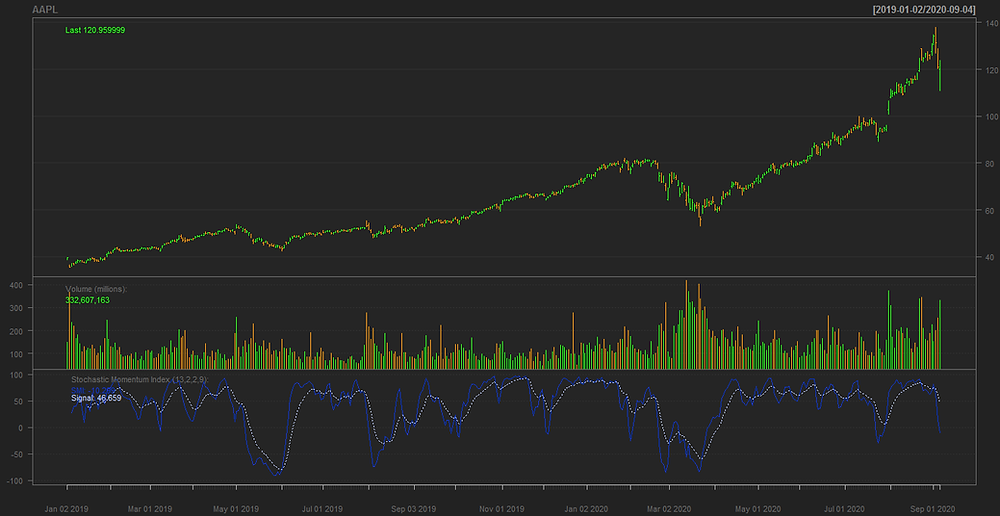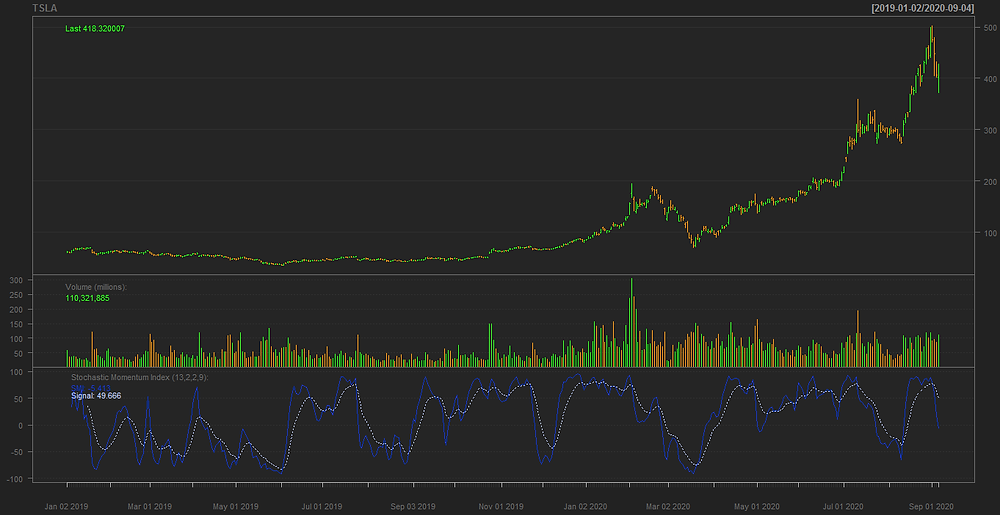Williams %R

To calculate Williams %R, we have to pass on the daily High, Low, and Close prices of companies along with a specified number of periods. In this step, we are going to take 14 days as the number of periods.

The following code will calculate the companies' Williams %R along with a plot:### Step-4: Creating Trading Signals

In this step, we are going to use the previously created indicators to build 'BUY' or 'SELL' trading signals. Basically, we will pass on specific conditions and if the condition satisfies a 'BUY' signal our created trading signal will turn to 1 which means a buy. If the condition satisfies a 'SELL' signal our created trading signal will turn to -1 which means a sell. If it doesn't satisfy any of the mentioned conditions, then it will turn to 0 which means nothing. It might sound like a whole bunch of fuzz but, it will be clear once we dive into the coding section. After coding your trading signals, you can use the 'which' command in R to see how many 'BUY' signals and how many 'SELL' signals we've got from our given condition.

Simple Moving Average

The following code will create SMA trading signals for companies if it satisfies our given conditions:

Parabolic Stop and Reverse (SAR)

The following code will create Parabolic SAR trading signals for companies if it satisfies our conditions:

Commodity Channel Index (CCI)

The following code will create CCI trading signals for companies if it satisfies our conditions:

Rate of Change (ROC)

The following code will create Rate Of Change (ROC) trading signals for companies if it satisfies our conditions:

Stochastic Momentum Index (SMI)

The following code will create the Stochastic Momentum Index (SMI) trading signals for companies if it satisfies our conditions:

Williams %R

The following code will create Williams %R trading signals for companies if it satisfies our conditions: